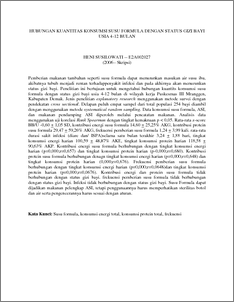# HUBUNGAN KUANTITAS KONSUMSI SUSU FORMULA DENGAN STATUS GIZI BAYI USIA 4-12 BULAN

SUSILOWATI, HENI (2006) HUBUNGAN KUANTITAS KONSUMSI SUSU FORMULA DENGAN STATUS GIZI BAYI USIA 4-12 BULAN. Undergraduate thesis, Diponegoro University.Preview
PDF - Published Version
15Kb

Official URL: http://www.fkm.undip.ac.id

## Abstract

Pemberian makanan tambahan seperti susu formula dapat menurunkan masukan air susu ibu, akibatnya tubuh menjadi rentan terhadappenyakit infeksi dan pada akhirnya akan menurunkan status gizi bayi. Penelitian ini bertujuan untuk mengetahui hubungan kuantits konsumsi susu formula dengan status gizi bayi usia 4-12 bulan di wilayah kerja Puskesmas III Mranggen, Kabupaten Demak. Jenis penelitian explanatory research menggunakan metode survei dengan pendekatan cross sectional. Delapan puluh empat sampel dari total populasi 254 bayi diambil dengan menggunakan metode systematical random sampling. Data konsumsi susu formula, ASI, dan makanan pendamping ASI diperoleh melalui pencatatan makanan. Analisis data menggunakan uji korelasi Rank Spearman dengan tingkat kemaknaan p < 0,05. Rata-rata z-score BB/U -0,60 + 1,05 SD, kontribusi energi susu formula 14,60 + 25,25% AKG, kontribusi protein susu formula 29,47 + 59,26% AKG, frekuensi pemberian susu formula 1,24 + 3,99 kali. rata-rata durasi sakit infeksi (diare dan/ ISPA)selama satu bulan terakhir 3,24 + 1,88 hari, tingkat konsumsi energi harian 100,59 + 48,87% AKE, tingkat konsumsi protein harian 118,58 + 90,63% AKP. Kontribusi energi susu formula berhubungan dengan tingkat konsumsi energi harian (p=0,000;r=0,657) dan tingkat konsumsi protein harian (p-0,000;r=0,680). Kontribusi protein susu formula berhubungan denagn tingkat konsumsi energi harian (p=0,000;r=0,648) dan tingkat konsumsi protein harian (0,000;r=0,676). Frekuensi pemberian susu formula berhubungan dengan tingkat konsumsi energi harian (p=0,000;r=0,0648)dan tingkat konsumsi protein harian (p=0,000;r=0,0676). Kontribusi energi dan protein susu formula tidak berhubungan dengan status gizi bayi. frekuensi pemberian susu formula tidak berhubungan dengan status gizi bayi. Infeksi tidak berhubungan dengan status gizi bayi. Susu Formula dapat dijadikan makanan pelengkap ASI, tetapi penggunaannya harus memperhatikan sterilitas botol dan air serta pengencerannya harus sesuai dengan aturan. Kata Kunci: Susu formula, konsumsi energi total, konsumsi protein total, frekuensi CORRELATION BETWEEN QUANTITY OF INFANT FORMULA FEEDING AND THE NUTRITIONAL STATUS AMONG INFANTS AGED FOUR TO TWELVE MONTHS Formula feeding to breastfed infants may reduce breastmilk. Further, formula feeding will also increase the susceptibility to infection, which in turn leads to decreased nutritional status of the infants. The aims of the research was to correlate the quantity of formula milk consumed with the nutritional status of the infants aged four to twelve months, who lived in the working area of Puskesmas III Mranggen, Demak. This explanatory study used survey method with a cross sectional approach. Eighty-four samples were selected by systematic random sampling method from 254 children in the population. Data on the quantity and frequency of the formula consumed as well as breastmilk and other food were obtained from the obtained the mothers using recall method. Data analysis was conducted using Rank Spearman correlation test. P-values less than 0.05 were consideredas significant. The mean WAZ score of the infants was -0.06 + 1.05 SD, Infant formula contributed to 14.60 + 25.25% RDA for energy, 29.47 + 59.26% RDA for protein. The frequency of formula intake was 1.24 + 1.88 times per day and the mean duration of infection (diarrhoea and respiratory infection) during the last month before the study was 3.24 + 3.99 days. The mean total intake for energy per day was 100.59 + 48.87% RDA and for protein was 118.58 + 90.63% RDA. There were correlations between formula contribution to energy intake with the total energy intake (p=0.000;r=0.657) and total protein intake (p=0.000;r=0.680). there were correlations between formula contribution to protein intake with the total energy intake (p=0.000;r=0.676) and total protein intake (p=0.000;r=0.676). there were correlations between formula feeding frequency with the total energy intake (p=0.000;r=0.648)and total protein intake (p=0.000;r=0.676). There was no correlation between formula contribution to energy intake, protein intake feeding frequency of infant formula with WAZ scores of the infants. There was no correlation between the duration of infections and WAZ scores of the infants. Infant formula can be as an alternatif of breastmilk supplement food, but the function should pay attention to the sterility of bottle and water and also the dilutation should based on the rule Keyword: infant formula, total energy intake, total protein intake, feeding frequency

Item Type: Thesis (Undergraduate) R Medicine > RA Public aspects of medicine > RA0421 Public health. Hygiene. Preventive Medicine Faculty of Public Health > Department of Public Health 9161 INVALID USER 23 Apr 2010 10:30 23 Apr 2010 10:30

Repository Staff Only: item control page